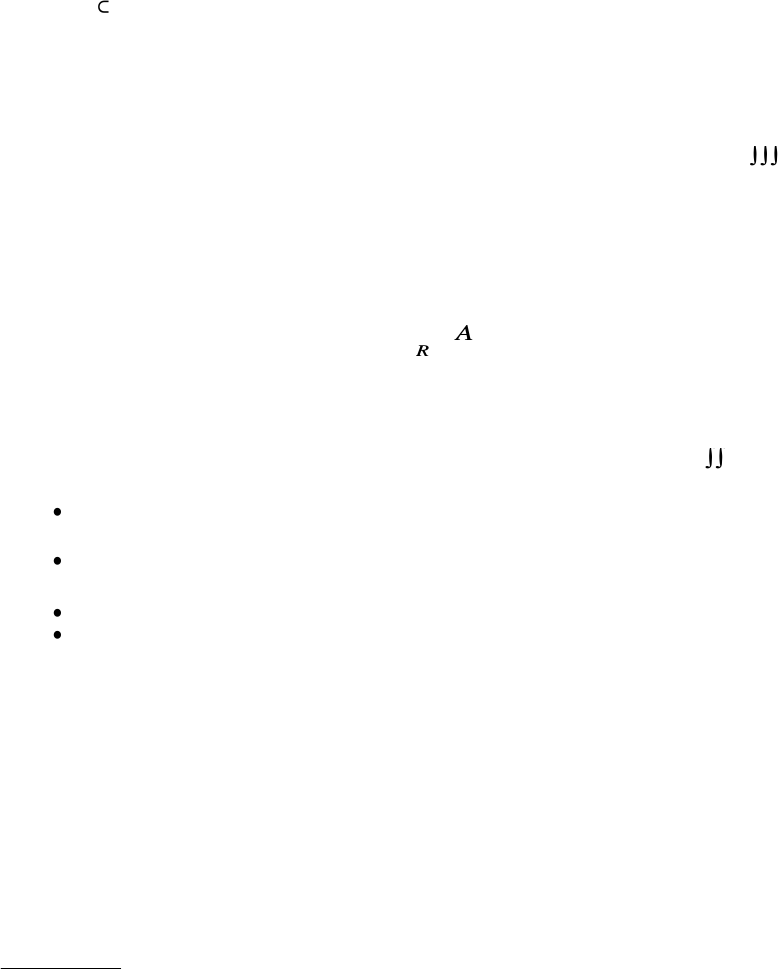Study Guides (380,000)
CA (150,000)
UTSG (10,000)
MAT (1,000)
Final

MAT237Y1 Final: Notes10Exam

Department
Mathematics
Course Code
MAT237Y1
Professor
John Bland
Study Guide
Final

This preview shows pages 1-2. to view the full 7 pages of the document.
2.1.
abstract theory of integration for functions of a single variable. The lectures
covered most of Section 4.1 of Folland, but omitting some details of proofs, and largely
skipping the Fundamental Theorem of Calculus (because
we assume that you remember
the statement and how to use it
, and we did not want to go into the proof.)
This was mostly review from MAT137, and the main points here all reappear in our
discussion of integration in higher dimensions.
To refresh your memory, you may want to review the nice series of videos from MAT137
that go over the definition of the integral in 1 dimension. (Search for definition of integral
One of the concepts that appears here and that you may not have seen in MAT137
is the notion of
zero
content. This is important for integration in higher dimensions,
particularly when we want to integrate over any set
S
that is not a rectangle. Then
zero
content can help us determine whether or not this is possible. One reason we study zero
content in 1 dimension is to help us get a grip on the concept for higher dimensions, where
it is really useful.
2.2.
Integration of functions of two or more variables.
2.2.1.
main practical conclusions.
For practical purposes, the most important conclusion
from the abstract theory of integration in
R
2
is that it makes sense to talk about integrals
of the form
whenever
∫∫
S
f
dA
1

Only pages 1-2 are available for preview. Some parts have been intentionally blurred.2
∫∫∫
∫∫
×
fdA
S
R
2
is a bounded set whose boundary consists of one or more smooth curves,
and
f
is
con
tin
uous
on
S
¯
(whic
h
implies
in
particular
that
f
is
b
ounded.)
.
These are far from the most general possible conditions, but in practice, this is normally
the kind of integration problem we are interested in, particularly in MAT237.
Similar considerations apply in higher dimensions, although we did not discuss everything
in complete detail. But for example in 3d, it is always possible to talk about
S
f
dV
if
S
R
3
is
a
union
of
smo
oth
surfaces
and
f
is
con
tin
uous
on
S
¯
.
2.2.2.
main theoretical points.
You are responsible for knowing1
the definition of the integral
∫∫
where
R
is a rectangle in
R
2
and
f
is a function defined on
R
. This includes the
definitions of
upper and lower Riemann sums
upper and lower Roiemann integral
integrability, and (when a function is integrable) the integral
f
dA
.
basic properties of integration; see Theorem 4.17 in Follands
,
Section 4.2.
The definition of
zero content
, and basic properties of zero content, see Proposition
4.19 in Follands
, Section 4.2.
The definition of a measurable set.
Sufficient conditions for a function
f
to be integrable on a set
S
. SeeTheorem
4.21 in Follands
, Section 4.2. This is a more general and more
complicated version of the main practical conclusion summarized in Section 2.2.1
above of these notes.
2.3.
Iterated integrals.
In practice, one
almost never
computes a integral straight from
the definition involving Riemann sums. Instead, we reduce the problem of integration to a
problem of carrying out repeated 1-dimensional integrals, which we can do using techniques
from single-variable calculus.
The main fact is that if
R
=
[
a, b
] [
c, d
] is a rectangle in
R
2
, then under suitable
hypotheses,
∫∫
f
dA
=
d
.
b
f
(
x, y
)
dx
Σ
dy
R c a
1this means: first make sure that you know the basic material the definitions and the main theorems
etc then make sure that you are able to use this material to solve problems. Should you memorize proofs?
Not unless
you are completely sure that you know the basic material and can use the it to solve problems.
you understand the theorems well enough to
extract what you consider to be the main steps/main ideas of the proofs,
remember only these, and
reconstruct the proofs from your memory of the main steps.
R
You're Reading a Preview

Unlock to view full version